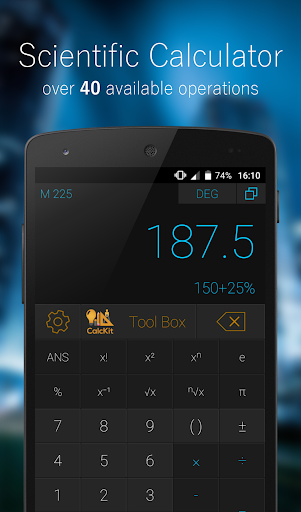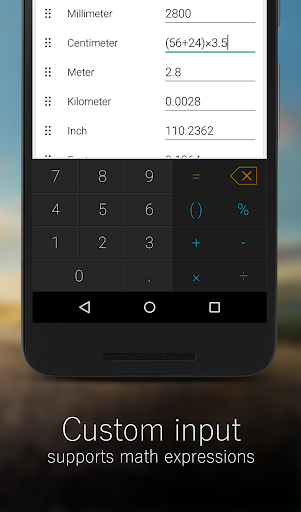Có gì mới?

# CalcKit: All in One Calculator Premium

• Không có

#### china

CalcKit: All in One Calculator is a lightweight, clean and easy to use all in one package with over 150 unique calculators and converters.

Containing a large collection of unit converter, electronic and mathematic calculators, packed in with a beautifully designed Scientific Calculator, it's the only calculator app you will ever need on your device.

It contains great calculators for school and homework, but not only. If you're a scholar, student, teacher, handyman, enthusiast, engineer... and you need an all in one calculator for your device, give CalcKit a try.

CalcKit features:
✓ Scientific & RPN Calculators
✓ Floating Scientific & RPN Calculators
✓ 44 Mathematic Calculators
✓ 21 Electronic Calculators
✓ 81 Unit Converters
✓ 3 Financial Calculators
✓ Custom Tools Creator with up to 25 Variables
✓ Supports math expressions as input
✓ Integrated Search
✓ Favorites List for quick access
✓ Light & Dark Calculator Themes
✓ 6 Languages

Languages: English, French, German, Greek, Spanish, Macedonian

Complete list of all calculators and unit converters
Geometry

• Triangle
• Right Triangle
• Square
• Rectangle
• Parallelogram
• Rhombus
• Trapezoid
• Isosceles Trapezoid
• Regular Hexagon
• Regular Polygon
• Circle
• Segment and Sector of Circle
• Ellipse
• Cube
• Cuboid
• Regular Prism
• Pyramid
• Pyramidal Frustum
• Regular Tetrahedron
• Regular Octahedron
• Cylinder
• Cylinder with an oblique plane face
• Cone
• Frustum of Cone
• Sphere
• Spherical Cap, Sector, Segment and Wedge
• Torus
Equations
• Linear, Quadratic and Cubic Equations
• 2x2 and 3x3 System
Analytical Geometry
• Distance between 2 points
• Area of Triangle
• Equation of Circle and Sphere
Algebra
• Prime Number Check
• Proportion Calculator
• Decimal to Fraction
• Fraction Simplifier
• Prime Factorization
• GCF / LCM
Matrix Calculator
• Determinant
• Inverse
• Transpose
• A × B
• A + B
• A - B
Electronics
• Resistor and Inductor Color Codes
• Series/Parallel Components
• Ohms Law
• Power Triangle
• Y - Δ Transformation
• Voltage Regulator
• NE555 Timer
• Reactance
• Battery Life Calculator
• Wire Resistivity
• Transformer Ratio
• Analog - Digital Converter
• Operational Amplifier
• Filters
• LED Resistor Calculator
• Frequency Calculator
• Voltage Divider
Finance
• Currency Converter
• VAT / Tax Calculator
• Tip Calculator
Common Converters
• Numbers
• Roman Numerals
• Prefixes
• Angle
• Area
• Cooking
• Data Storage
• Fuel Consumption
• Mass / Weight
• Length / Distance
• Temperature
• Time
• Speed
• Pressure
• Power
• Volume
• Force
• Energy / Work
• Absorbed Dose
• Activity
• Exposure
Magnetism Converters
• Magnetic Field Strength
• Magnetic Flux and Flux Density
• Magnetomotive Force
Light Converters
• Illumination, Luminance and Luminous Intensity
Engineering Converters
• Acceleration
• Acceleration - Angular
• Airflow
• Density
• Moment of Force and Inetria
• Specific Volume
• Torque
• Velocity - Angular
Electricity Converters
• Charge
• Current
• Conductance
• Conductivity
• Field Strength
• Potential
• Resistance
• Resistivity
• Capacitance
• Inductance
• Linear Charge Density
• Linear Current Density
• Surface Charge Density
• Surface Current Density
• Volume Charge Density
Fluids Converters
• Concentration - Molar and Solution
• Flow
• Flow - Mass
• Flow - Molar
• Mass Flux Density
• Permeability
• Surface Tension
• Dynamic Viscosity
• Kinematic Viscosity
Heat Converters
• Fuel Efficiency - Mass
• Fuel Efficiency - Volume
• Heat Density
• Heat Flux Density
• Heat Transfer Coefficient
• Specific Heat Capacity
• Temperature Interval
• Thermal Conductivity, Expansion and Resistance
Other Converters
• Frequency
• Image Resolution
• Pace
• Pixel Density
• Sound
• Typography
• Volume - Dry
• Volume - Lumber